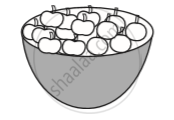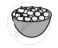Share

# Recalling Ratios and Percentages

Course

#### notes

Ratio means comparing two quantities.
A basket has two types of fruits, say, 20 apples and 5 oranges.Then, the ratio of the number of oranges to the number of apples = 5 : 20.
The comparison can be done by using fractions as , 5/20 = 1/4
The number of oranges is 1/4th the number of apples. In terms of ratio, this is 1 : 4, read as, “1 is to 4”  OR
Number of apples to number of oranges = 20/5 = 4/1 = which means, the number of apples is  4 times the number of oranges. This comparison can also be done using percentages.
There are 5 oranges out of 25 fruits. So percentage of oranges is 5/25 xx 4/4 = 20/100 = 20%  [Denominator made 100].   OR

By unitary method:   Out of 25 fruits, number of oranges are 5. So out of 100 fruits, number of oranges  =5/25 xx 100 = 20
Sincecontains only apples and oranges ,

So, percentage of apples + percentage of oranges = 100
or percentage of apples + 20 = 100
or percentage of apples = 100 – 20 = 80
Thus the basket has 20% oranges and 80% apples.

If you would like to contribute notes or other learning material, please submit them using the button below.

### Shaalaa.com

Recalling Ratios and Percentages [00:09:27]
S
0%